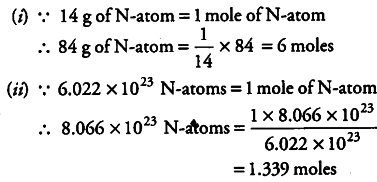# Calculate the number of moles for the following:84 g of nitrogen atoms

Calculate the number of moles for the following:
(i) 84 g of nitrogen atoms
(ii) 8.066 x \${10}^{23}\$ number of N-atoms
[Atomic mass of N = 14]